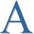PowerTip: Round a Number with PowerShellDr Scripto

Summary: Use Windows PowerShell to round numbers to a specific decimal place.How can I use Windows PowerShell to round a number to a specific number of decimal places, and continue to
have a number instead of converting it to a string.Use the Round static method from the System.Math class. By default, it does not display decimal places, but
you can easily change that behavior. The following code illustrates this procedure:

PS C:\> \$a = 111.2226

PS C:\> [math]::Round(\$a)

111

PS C:\> [math]::Round(\$a,2)

111.22

PS C:\> [math]::Round(\$a,3)

111.223